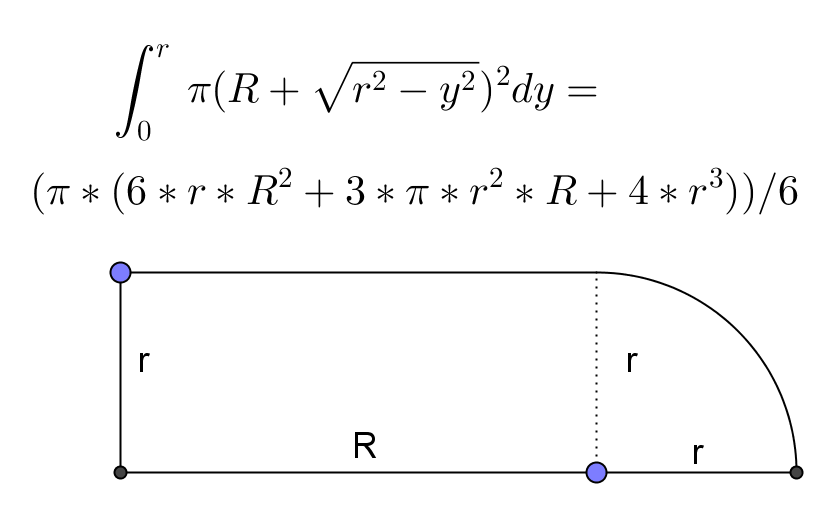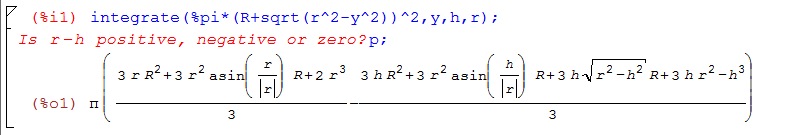# Volume of revolution of an irregular shape

Forest_roby shared this question 7 years ago

Hi!

Is it possible for geogebra to calculate volume of revolution of a drawing ( 2d drawing of a mechanical part ) not function like f(x)=x^2 or anything like that (not easy to integrate it)? I calculated the volume with autocad but if it is possible with geogebra I would be very happy. I want to modify the drawing according to different parts of volume - and that is very hard with autocad - at least for me.

I attached an example document in which I am interested about volumes of some parts rotated around y axis.

Thanks for all the help!1

hellosaludos1

I should have been more specific. Well I am interested about the volume of many parts, in the attached doc:

(Q1, O1, I1, H1, F1) or

(T, D1, Q1, P1, N,L)

And to be even more specific - I want to modify in the drawing and see how the volumes change. It is not a finished drawing, there are point and areas missing but I thought it is a good start.1

hello

you can also to use an integral formula for each shape similar I said in first post

example for (Q1, O1, I1, H1, F1)being R=x(I_1)-x(F_1) this easier if you create the segment because R is the value of segment

h=y(L_1)-y(F_1)

you can define a function f(x,y,z) like the screenshoot of maxima

then f(R,r,h) says the volumen

saludos

saludos1

It took me some time to answer you back cos I wanted to check what you wrote here. I do not believe that your integrate is correct but!

But now I know that geogebra can not calculate the volume on its own. I made an integral - actually I made two to count the volume and it works - I used the maxima program as well, thanks for that too.

I made the integrals on paper but I used geogebra to calculate the function of circle. (the center point of the radius is not at all obvious and very important) -->attached file

Now I have to figure out the integral for the other volumes I want and put it in a dinamic geogebra file where I can modify the volumes. - Needle to say, I will be back as soon as I have time. :)

Thanks!1